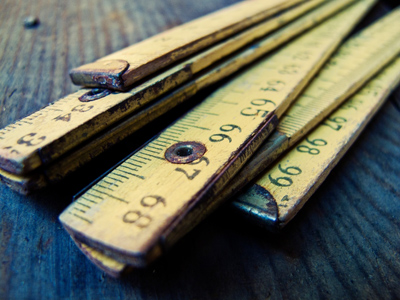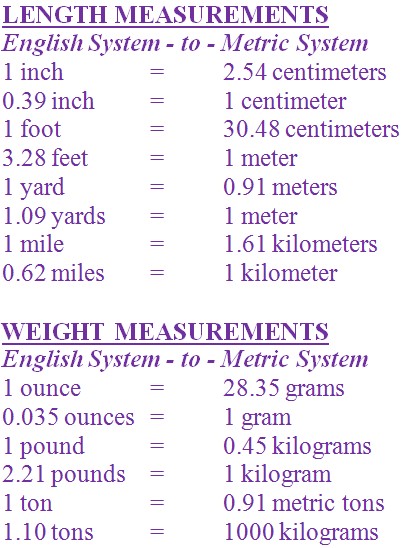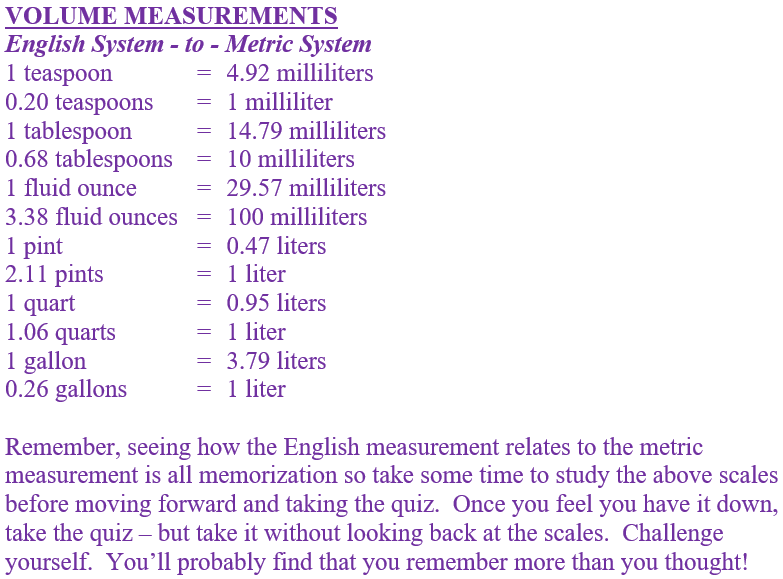See how much you remember about the metric system in this quiz.

# Measurement - Metric System/English System Conversion Scale

This Math quiz is called 'Measurement - Metric System/English System Conversion Scale' and it has been written by teachers to help you if you are studying the subject at middle school. Playing educational quizzes is a fabulous way to learn if you are in the 6th, 7th or 8th grade - aged 11 to 14.

It costs only \$12.50 per month to play this quiz and over 3,500 others that help you with your school work. You can subscribe on the page at Join Us

In your math lessons you learned that in the U.S. we use what is called the American system of measurement or the English system of measurement whereas most of the rest of the world uses the metric system.

The metric system is truly an easier system in that it works in bases of 10. However, for Americans who are trying to learn the metric system, they tend to want to compare the English system with the metric system. For example, if someone wants to buy a gallon of milk, they wonder what that would equal to in the metric system. (Remember - this would be measuring milk in liters.) The answer is - it doesn’t - at least not exactly. Why? Because the English system does not work in the base of 10 so there is no “exact” equivalent to the metric system.

What does this all mean? When trying to “compare” or relate one system to the other - sheer “memorization” is involved. There is no set formula that converts the metric system to the English system or vice versa. Still “people want to know.” Therefore, below are a few conversion scales that might help you to understand or visualize how one measurement system compares to another measurement system. Take a look below for more detail.

Go straight to Quiz1.
1 centimeter is equal to ____ inches.
0.28
0.39
0.29
0.38
1 centimeter is equal to 0.39 inches. Answer (b) is the correct answer
2.
0.62 miles is equal to _______.
1 kilometer
1 meter
1 millimeter
1 centimeter
0.62 miles is equal to 1 kilometer. Answer (a) is the correct answer
3.
1 pound is equal to ______ kilograms.
0.045
4.5
0.45
0.0045
1 pound is equal to 0.45 kilograms. Answer (c) is the correct answer
4.
4.92 milliliters is equal to _____.
1 tablespoon
1 pint
1 teaspoon
1 ounce
4.92 milliliters is equal to 1 teaspoon. Answer (c) is the correct answer
5.
3.28 feet is equal to ______ meter(s).
1.09 meters
1 meter
10 meters
0.1 meters
3.28 feet is equal to 1 meter. Answer (b) is the correct answer
6.
1 mile is equal to ______ kilometers.
1.061
1.16
6.11
1.61
7.
1 pint is equal to _____ liters.
7.04
4.07
0.47
4.70
8.
30.48 centimeters is equal to ______.
1 mile
1 inch
1 yard
1 foot
30.48 centimeters is equal to 1 foot. Answer (d) is the correct answer
9.
1 gallon is equal to _____ liters.
2.97
5.97
4.97
3.79